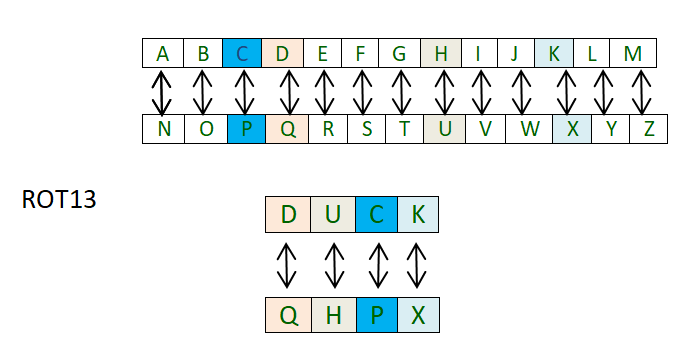Open in App
Not now

# ROT13 cipher

• Difficulty Level : Basic
• Last Updated : 12 Jul, 2022

ROT13 cipher(read as – “rotate by 13 places”) is a special case of the Ceaser cipher in which the shift is always 13.
So every letter is shifted 13 places to encrypt or to decrypt the message.You must think that it is just another caesar cipher so what’s different this time? Well the difference is in its implementation. The approach is to use two separate python dictionaries.

1. First one to lookup the various letters according to their place in the English alphabets to get the shifted number
2. Second one to get the letters which correspond to those shifted numbers.

Implementation:

## C++

 `// CPP program to implement``// ROT13 Caesar Cipher` `#include``using` `namespace` `std;` `// Map to lookup the index of alphabets``map <``char``,``int``> dict1;` `// Map to lookup alphabets corresponding``// to the index after shift``map <``int``,``char``> dict2;` `// Function to create map to lookup``void` `create_dict()``{``    ``for``(``int` `i = 1; i < 27; i++)``        ``dict1[``char``(64 + i)] = i;``   ` `    ``dict2 = ``'Z'``;``    ` `    ``for``(``int` `i = 1; i < 26; i++)``        ``dict2[i] = ``char``(64 + i);``        ` `    ``return``;``}` `// Function to encrypt the string``// according to the shift provided``string encrypt(string message, ``int` `shift)``{``    ``string cipher = ``""``;``    ``for``(``int` `i = 0; i < message.size(); i++)``    ``{``        ``// Checking for namespace``        ``if``(message[i] != ``' '``)``        ``{``            ``// looks up the map and``            ``// adds the shift to the index``            ``int` `num = (dict1[message[i]] + shift) % 26;``            ` `            ``// looks up the second map for the``            ``// shifted alphabets and adds them``            ``cipher += dict2[num];``        ``}``        ``else``        ``{``            ``// adds space``            ``cipher += ``" "``;``        ``}``    ``}``    ``return` `cipher;``}` `// Function to decrypt the string``// according to the shift provided``string decrypt(string message, ``int` `shift)``{``    ``string decipher = ``""``;``    ``for``(``int` `i = 0; i < message.size(); i++)``    ``{``        ``// checks for space``        ``if``(message[i] != ``' '``)``        ``{``            ``// looks up the map and``            ``// subtracts the shift to the index``            ``int` `num = (dict1[message[i]] - shift + 26) % 26;``            ``// looks up the second map for the``            ``// shifted alphabets and adds them``            ``decipher += dict2[num];``        ``}``        ``else``        ``{``            ``// adds space``            ``decipher += ``" "``;``        ``}``    ``}``    ``return` `decipher;``}` `// Driver code``int` `main()``{``    ``create_dict();``    ` `    ``string message = ``"GEEKS FOR GEEKS"``;``    ``int` `shift = 13;``    ` `    ``cout << encrypt(message, shift) << ``"\n"``;``    ` `    ``message = ``"TRRXF SBE TRRXF"``;``    ``shift = 13;``    ` `    ``cout << decrypt(message, shift) << ``"\n"``;``    ` `    ``return` `0;``}``// This code is contributed by Sachin Bisht`

## Python3

 `# Python program to implement``# ROT13 Caesar cipher` `'''This script uses dictionaries instead of 'chr()' & 'ord()' function'''` `# Dictionary to lookup the index of alphabets``dict1 ``=` `{``'A'` `: ``1``, ``'B'` `: ``2``, ``'C'` `: ``3``, ``'D'` `: ``4``, ``'E'` `: ``5``,``        ``'F'` `: ``6``, ``'G'` `: ``7``, ``'H'` `: ``8``, ``'I'` `: ``9``, ``'J'` `: ``10``,``        ``'K'` `: ``11``, ``'L'` `: ``12``, ``'M'` `: ``13``, ``'N'` `: ``14``, ``'O'` `: ``15``,``        ``'P'` `: ``16``, ``'Q'` `: ``17``, ``'R'` `: ``18``, ``'S'` `: ``19``, ``'T'` `: ``20``,``        ``'U'` `: ``21``, ``'V'` `: ``22``, ``'W'` `: ``23``, ``'X'` `: ``24``, ``'Y'` `: ``25``, ``'Z'` `: ``26``}` `# Dictionary to lookup alphabets``# corresponding to the index after shift``dict2 ``=` `{``0` `: ``'Z'``, ``1` `: ``'A'``, ``2` `: ``'B'``, ``3` `: ``'C'``, ``4` `: ``'D'``, ``5` `: ``'E'``,``        ``6` `: ``'F'``, ``7` `: ``'G'``, ``8` `: ``'H'``, ``9` `: ``'I'``, ``10` `: ``'J'``,``        ``11` `: ``'K'``, ``12` `: ``'L'``, ``13` `: ``'M'``, ``14` `: ``'N'``, ``15` `: ``'O'``,``        ``16` `: ``'P'``, ``17` `: ``'Q'``, ``18` `: ``'R'``, ``19` `: ``'S'``, ``20` `: ``'T'``,``        ``21` `: ``'U'``, ``22` `: ``'V'``, ``23` `: ``'W'``, ``24` `: ``'X'``, ``25` `: ``'Y'``}` `# Function to encrypt the string``# according to the shift provided``def` `encrypt(message, shift):``    ``cipher ``=` `''``    ``for` `letter ``in` `message:``        ``# checking for space``        ``if``(letter !``=` `' '``):``            ``# looks up the dictionary and``            ``# adds the shift to the index``            ``num ``=` `( dict1[letter] ``+` `shift ) ``%` `26``            ``# looks up the second dictionary for``            ``# the shifted alphabets and adds them``            ``cipher ``+``=` `dict2[num]``        ``else``:``            ``# adds space``            ``cipher ``+``=` `' '` `    ``return` `cipher` `# Function to decrypt the string``# according to the shift provided``def` `decrypt(message, shift):``    ``decipher ``=` `''``    ``for` `letter ``in` `message:``        ``# checks for space``        ``if``(letter !``=` `' '``):``            ``# looks up the dictionary and``            ``# subtracts the shift to the index``            ``num ``=` `( dict1[letter] ``-` `shift ``+` `26``) ``%` `26``            ``# looks up the second dictionary for the``            ``# shifted alphabets and adds them``            ``decipher ``+``=` `dict2[num]``        ``else``:``            ``# adds space``            ``decipher ``+``=` `' '` `    ``return` `decipher` `# driver function to run the program``def` `main():``    ``# use 'upper()' function to convert any lowercase characters to uppercase``    ``message ``=` `"GEEKS FOR GEEKS"``    ``shift ``=` `13``    ``result ``=` `encrypt(message.upper(), shift)``    ``print` `(result)` `    ``message ``=` `"TRRXF SBE TRRXF"``    ``shift ``=` `13``    ``result ``=` `decrypt(message.upper(), shift)``    ``print` `(result)` `# Executes the main function``if` `__name__ ``=``=` `'__main__'``:``    ``main()`

Output

```TRRXF SBE TRRXF
GEEKS FOR GEEKS```

Analysis: The ROT13 cipher is not very secure as it is just a special case of the Caesar cipher. The Caesar cipher can be broken by either frequency analysis or by just trying out all 25 keys whereas the ROT13 cipher can be broken by just shifting the letters 13 places. Therefore it has no practical use.

Application: ROT13 was in use in the net.jokes newsgroup by the early 1980s.

This article is contributed by Palash Nigam . If you like GeeksforGeeks and would like to contribute, you can also write an article using contribute.geeksforgeeks.org or mail your article to contribute@geeksforgeeks.org. See your article appearing on the GeeksforGeeks main page and help other Geeks.

My Personal Notes arrow_drop_up